# Test Case

## Brief Description of the Study Test Case

A 3D sketch of the experimental setup is shown in Fig. 1. The configuration presents a Glauert-Goldschmied type body consisting of a relatively long fore body and a relatively short concave ramp comprising the aft part of the model mounted between two glass endplate frames with both leading edge and trailing edges faired smoothly with a wind tunnel splitter plate.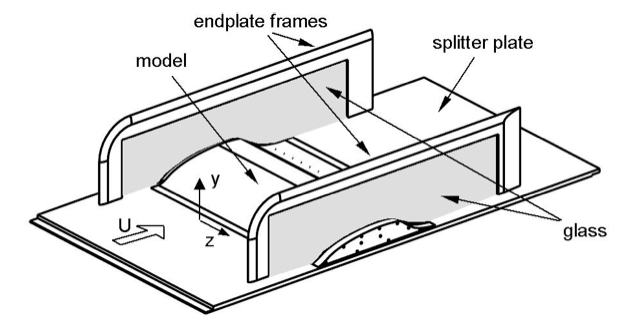Figure 1: 3D sketch of experimental setup [‌1], [‌2]

Major geometric and flow parameters of the TC are presented in Fig. 2 and summarized in Table 1 (note that in the “baseline” experiment considered here the slot shown in Fig. 2 was closed).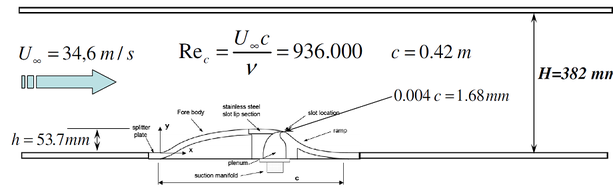Figure 2: Schematic of TC geometry and flow parameters

Table 1: Major geometrical and flow parameters
Parameter Notation Value
Free stream velocity ${U_{\infty }}$34.6 m/s
Hump chord ${c}$0.42 m
Crest height ${h}$0.0537 m
Reynolds number ${{\text{Re}}=U_{\infty }c/\nu }$936,000
Mach number ${\text{M}}$0.1

The experimental data are available at http://cfdval2004.larc.nasa.gov/case3expdata.html (Case 3), at https://turbmodels.larc.nasa.gov/nasahump_val.html, and also enter the ERCOFTAC Classic Collection http://cfd.mace.manchester.ac.uk/ercoftac under number C.83. The data set includes: streamwise distributions of the surface pressure and skin-friction coefficients, ${C_{P}}$and ${C_{f}}$, and mean velocity and Reynolds stresses fields in the tunnel center-plane, roughly covering the region 0.63 < x/c < 1.39.

## Test Case Experiments

A photo of the experimental rig is presented in  Fig. 3.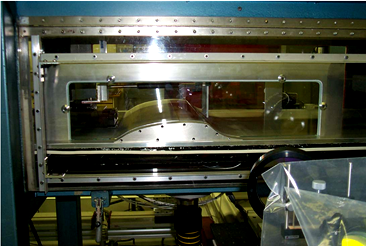Figure 3: Photo of the experimental rig

The experiments were performed in the NASA Langley 20′′×28′′ shear flow tunnel. The flow was nominally 2D, although with side-wall effects (3D flow) expected near the endplates. The reference “chord” length of the model, c, is defined as the length of the hump on the wall and is equal to 420mm. The maximum thickness of the hump, h, is equal to 53.7mm. The model was equipped with 153 centre-span static pressure ports and 20 dynamic pressure ports in the vicinity of the separated flow region. Sixteen spanwise pressure ports were located on the fore body (x/c = 0.19) and on the ramp at (x/c = 0.86). Two-dimensional PIV data were acquired in a plane, along the model centreline and normal to the surface, starting from right upstream of the slot and ending well beyond the reattachment location at x/c ≈ 1.4. Stereoscopic PIV (3D) data were acquired in planes perpendicular to the flow direction, arranged to intersect the 2D plane from x/c = 0.7 to 1.3 in steps of approximately 0.1. Oil-film interferometry was used to quantify the skin friction over the entire model, from the region upstream of the hump to beyond the reattachment location.

Two-dimensionality of the flow in the separated and reattachment regions was thoroughly assessed via three methods: by considering the spanwise pressures on the ramp in the separated region, performing 3D PIV measurements in planes perpendicular to the flow direction, and by means of the surface oil-film flow visualization. Spanwise distribution of the surface pressure was also measured on the fore body of the model. It was shown that the spanwise variations of the flow parameters are small (e.g., at the test condition, the pressure variation over the central half of the model (–0.25 ≤ z/c ≤ 0.25) is ∆CP = ±0.005) and that departures from twodimensionality are observed mainly near the wall.

At the inflow location (x/c = –2.14), pitot-probe and hot-wire anemometer data were compared with 2D and 3D PIV. Inflow skin friction was also documented using oil-film interferometry. Resulting inflow velocity profile which was used in the numerical studies as a benchmark for imposing boundary conditions at the inflow of the computational domain (see the next section) is shown in Fig. 4.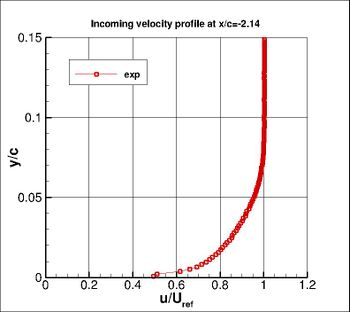Figure 4: Experimental profile of streamwise velocity at x/c = -2.14 (momentum thickness Reθ=7200)

Experimental uncertainties reported in the original experimental papers are summarized in Table 2. Note that there is also an operational uncertainty associated with the endplates blockage effect, which should be compensated in the quasi-2D simulations (see next sub-section).

Table 2: Experimental uncertainties
Quantity Experimental Uncertainty
Pressure coefficient, ${C_{\text{p}}}$± 0.001
Velocity Less than 3%
Reynolds shear stress Up to 20%
Root mean square of streamwise and wall-normal velocity fluctuations (normal Reynolds stresses) Up to 14%

## CFD Codes and Methods

As mentioned in the Introduction, the present document focuses on the three groups of CFD studies of the considered test case, namely, on its predictions obtained with the use of different RANS models [‌7], hybrid RANS-LES models [‌8], [‌9] and WRLES [‌6] (see Table 3).

Table 3: Summary of considered CFD studies
Source Turbulence Modelling approach
NASA Turbulence Modeling Resource Portal [‌7] RANS
Go4Hybrid EU Project [‌8], [‌9] Non-zonal and zonal RANS-LES hybrids
A. Uzun & M.R. Malik [‌6] Wall Resolved LES

Naturally, corresponding numerical procedures and codes are quite different.

In particular, the RANS based computations, results of which are presented on the NASA Turbulence Modeling Resource portal were carried out with the use of two long-standing compressible Navier-Stokes CFD codes developed at NASA Langley Research Center for solving 2D and 3D compressible flows on structured (CFL3D code https://cfl3d.larc.nasa.gov/) and unstructured (FUN3D code https://fun3d.larc.nasa.gov/) grids. The grids used in the computations are built according to the well-know guidelines for solving RANS equations (the grids are available on the portal [‌7]) and both codes return virtually identical results, thus suggesting code- and (indirectly) grid-independence of the obtained solutions.

The RANS-LES computations carried out in [‌8], [‌9] employed two enhanced hybrid models equipped with special tools for the “Grey-Area” Mitigation (GAM): a non-zonal method Delayed DES (DDES) [‌10] with Shear-Layer Adapted (SLA) subgrid length scale ΔSLA [‌11] and a zonal Improved DDES (IDDES) [‌12] combined with Synthetic Turbulence Generator (STG) [‌13] or Synthetic Eddy Method (SEM) [‌14], [‌15] for imposing unsteady boundary conditions at the RANS-IDDES interface. The shear-layer adapted subgrid length scale [‌11] replaces the length scale of the original DDES equal to the maximum grid spacing Δmax = max{Δx, Δy, Δz}. It accounts for strong anisotropy typical of the grids in the initial part of separated shear layers and the quasi-2D character of the flow in this region. This substitution results in a considerable reduction of the subgrid eddy-viscosity, thus ensuring a rapid development of 3D turbulence structures.

A matrix of the performed simulations and appropriate references to original publications which provide a detailed outline of the approaches used are presented in Table 4.

Table 4: Matrix of hybrid RANS-LES computations [‌6], [‌8], [‌9]
Hybrid Method GAM tool Location of RANS-LES interface CFD Code
Zonal SST-based IDDES  SEM ,  x/c = 0.5
x/c = -1.0
Compressible TAU code with hybrid LD2 scheme 
Zonal SST-based IDDES  STG  x/c = 0.5
x/c = -1.0
Incompressible branch of NTS code 
Non-zonal SST-based DDES  Shear-Layer Adapted length scale, ΔSLA  No interface Incompressible branch of NTS code 

The non-zonal simulation was conducted with the use of the well-established in-house finite- volume structured multi-block code NTS, whereas for the zonal ones, along with this code, an unstructured compressible finite-volume code TAU of DLR was employed.

The incompressible branch of the NTS code used in the simulations is based on the flux-difference splitting method of Rogers and Kwak [‌18]. The approximation of the inviscid fluxes in the code depends on the turbulence representation approach: in the zonal RANS-IDDES computations, it is a 3rd-order upwind-biased scheme in the RANS zone and a 4th-order central scheme in the WMLES zone, whereas for the non-zonal DDES the hybrid (weighted 3rd-order upwind-biased / 4th-order central) scheme [‌19] is used. The viscous fluxes are approximated with the 2nd-order central scheme. For the time integration, an implicit 2nd-order backward Euler scheme with sub-iterations is applied.

The TAU code employs a low-dissipation low-dispersion (LD2) scheme [‌16] which is based on the 2nd-order energy-conserving skew-symmetric convection operator combined with a minimal level of 4th-order artificial matrix dissipation for stabilization. The central flux terms employ an additional gradient extrapolation that effectively increases the discretization stencil and is used to reduce the dispersion error of the scheme. Both ingredients are essential in terms of turbulence resolution with the unstructured TAU code. Note that in the present zonal RANS-WMLES computations, the LD2 scheme is only active in the respective IDDES region downstream of the interface. The temporal discretization is based on an implicit dual-time stepping scheme which is also of 2nd-order accuracy.

All the hybrid simulations were performed in the same computational domain and on the same grid (“mandatory” grid in the Go4Hybrid project).

The computational domain in the XY-plane is shown in Fig. 5. Its size in the spanwise direction is equal to 0.4c (this has been proven sufficient to arrive at a span-independent solution within the ATAAC project  [‌4]). As recommended in  [‌7], the contour of the upper (slip) wall of the domain was modified (moved somewhat downwards in the area above the hump) in order to compensate the blockage effect of the endplates (see Fig. 1 above).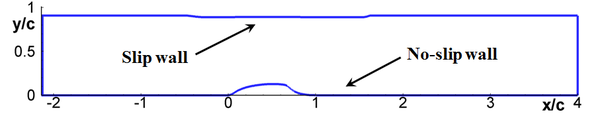Figure 5: Schematic of computational domain in XY-plane and wall boundary conditions , 

The inflow and outflow conditions in the simulations performed with the use of the TAU and NTS codes were somewhat different since the former code is compressible and in the latter one its incompressible branch was employed. In both cases velocity and turbulence quantities (k and ω) at the inflow boundary (x/c = -2.14) were specified from a precursor SST RANS of the zero pressure gradient boundary layer carried out to match the experimental value of the momentum thickness based Re-number ${{\text{Re}}_{\theta _{\text{inflow}}}=\theta _{\text{inflow}}U_{\infty }/\nu =7200}$(see Fig. 4 above). In the incompressible simulations (NTS code), the inflow static pressure was defined by the linear extrapolation of the values in the nearby interior cells of the domain, whereas in the compressible simulations (TAU code) flux boundary conditions were used together with an extrapolation of the pressure from the interior. At the outflow boundary, in the NTS code a constant static pressure is specified and all the other flow variables are defined by linear extrapolation from the interior cells of the domain, which is similar to TAU’s flux conditions with an imposed fixed exit pressure. Finally, in all simulations the boundary conditions in the spanwise direction were periodic.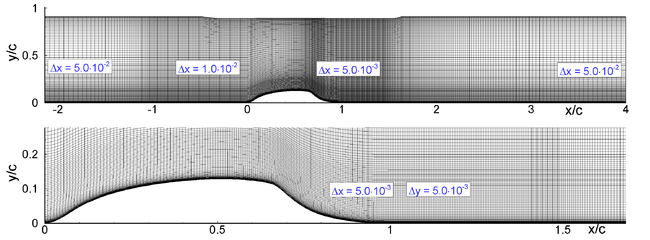Figure 6: Entire grid in XY-plane used in hybrid RANS-LES simulations and its zoomed-in view [‌8], [‌9]

The grid in the XY-plane used in all the simulations is shown in Fig. 6. It has 511×127 cells in the x- and y-directions, respectively. Near the bottom wall, the grid is wall-orthogonal and yields a first cell height in wall units (Δy1+) less than 1.0 in the whole domain. For -1.0 < x/c < 0.65, the grid is also refined in the x-direction in order to satisfy demands to WMLES grids in the outer flow units (Δx / δ < 0.1), which allows placing the RANS-LES interface at any cross-section within this region. In the separation (“focus”) region (0.67 < x / c < 1.5) both x- and maximum y-steps are equal to 0.005c. In the spanwise direction, the grid has 80 cells and is uniform with a step size equal to 0.005cz / δ < 0.05), thus resulting in a nearly cubic grid in the focus region. As a result, the total grid has around 5.2 million cells, that is, rather modest by today's standards. The physical time step is set to Δt = 0.002c / U which results in a convective CFL number of well below 0.5 in the focus region. The total simulation time was around 30 Convective Time Units, CTU (1 CTU = c / U), of which the last 20 CTUs were used for obtaining time-averaged flow data and turbulence statistics.

The last numerical database for the 2DWMH flow discussed in the present document is that accumulated in the course of the study [‌6] where compressible Wall Resolved LES of this flow has been performed (see Table 3). The code used in this study has been previously successfully applied to LES of different wall-bounded turbulent flows (see, e.g., [‌20] – [‌22]. It solves the unsteady fully three-dimensional compressible Navier-Stokes equations discretized on multi- block structured overset grids either with no SGS model (Implicit LES or ILES) or with the static SGS model of Vreman [‌23].

All the spatial derivatives in the code were computed employing an optimized pre-factored fourth-order compact finite-difference scheme [‌24], which offers improved dispersion characteristics. To eliminate spurious high-frequency oscillations that may arise from several sources (grid stretching, unresolved fluctuations, approximation of physical boundary conditions) and to ensure numerical stability, a sixth-order compact filtering scheme [‌25], [‌26] was employed. Finally, in order to maintain high-order accuracy throughout the entire computational domain, a sixth-order accurate explicit Lagrangian interpolation [‌27] was applied whenever overset grids were used. A Beam-Warming type approximately factorized implicit scheme was used for the time advancement (more details of the simulation methodology can be found in [‌20], [‌21], [‌28], [‌29]).

The simulations were performed in the same domain in XY-plane as used in the hybrid RANS-LES computations (see Fig. 5) with two different widths, one being the same as that in the hybrid simulations and the other two times less, i.e., 0.2c. The grid-spacings in the streamwise, spanwise, and wall-normal directions in the wall units Δx+ , Δz+, and Δy+in the near wall part of the boundary layer (up to about y+ ≈ 200) were equal to about 25, 12.5 and 0.8, respectively, and then the grid was coarsened by a factor of two in both the streamwise and spanwise directions. This was possible thanks to the solver overset-grid capability (a fragment of the two-level overset-grid in YZ-plane is shown in Fig. 7). As a result, the total grid had about 825 million points, which ensured resolving the boundary layer approaching the hump by 90 to 100 points and the thinnest part of the boundary layer over the hump by about 30 points in the wall-normal direction.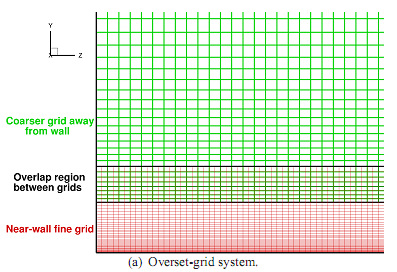Figure 7: Two-level overset-grid system at x/c = -2 

The time step of all simulations was 2.5×10-4c/aref (aref is the reference speed of sound) or 2.5×10-3c/Uref. Statistical results were averaged over about 10 CTU.

Similar to the hybrid simulations outlined above, the top boundary of the computational domain was treated as an inviscid wall while viscous adiabatic boundary conditions where imposed on the lower (hump) wall. In order to eliminate reflections from the outlet boundary, a sponge zone was placed downstream of the physical region of interest (x/c > 1.6). At the outflow boundary of the domain (x/c = 4) the back pressure was specified (set slightly below the upstream pressure) and characteristic boundary conditions were applied, whereas the inflow conditions were based on the characteristic relaxation boundary conditions [‌30] that inject turbulent fluctuations (generated by the inflow generation technique) in the boundary layer while allowing upstream- travelling waves to exit the domain.

Note finally that in the framework of WRLES several sensitivity tests were carried out aimed at finding out effects of the grid-resolution and span size of the computational domain and of the operational uncertainty associated with the blockage effect of end plates.

Contributed by: E. Guseva, M. Strelets — Peter the Great St. Petersburg Polytechnic University (SPbPU)